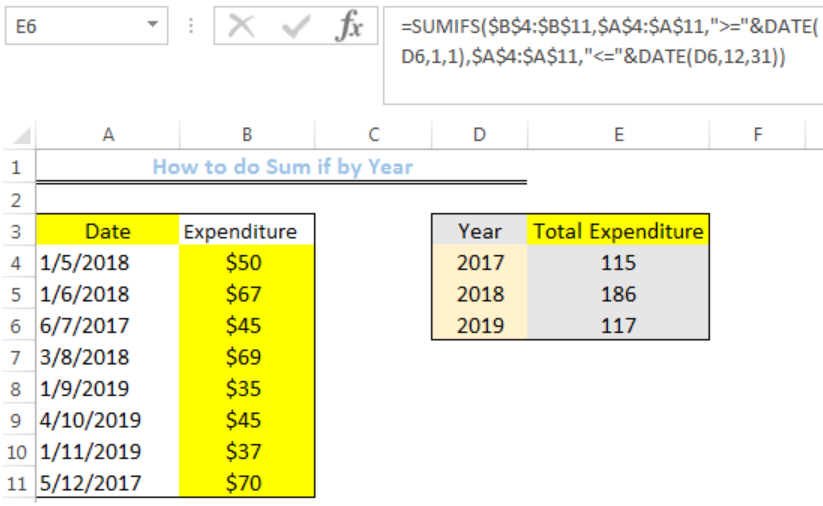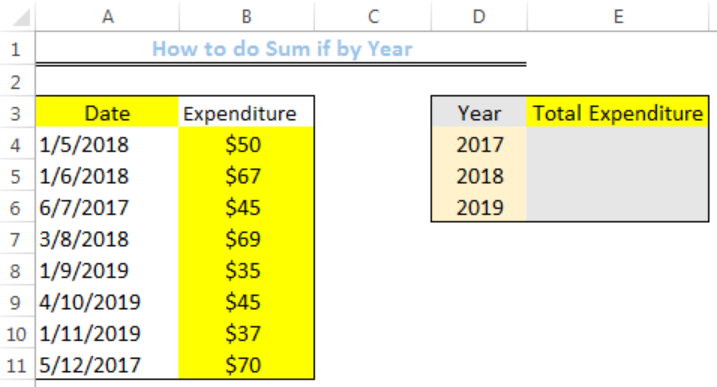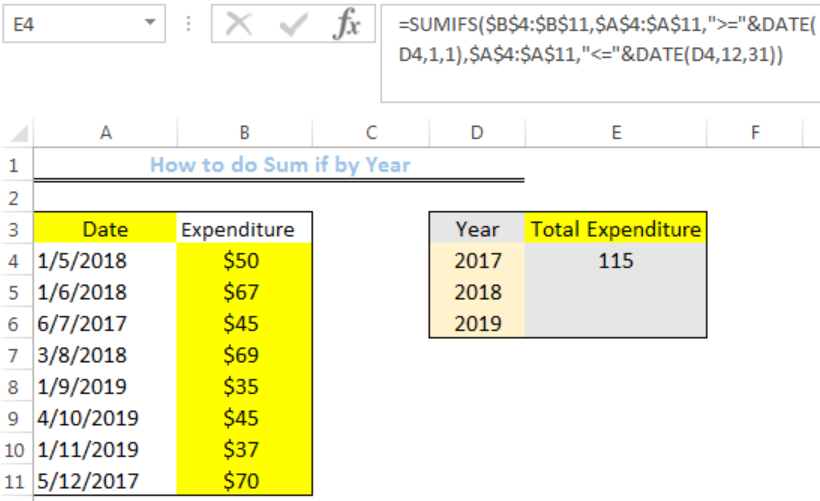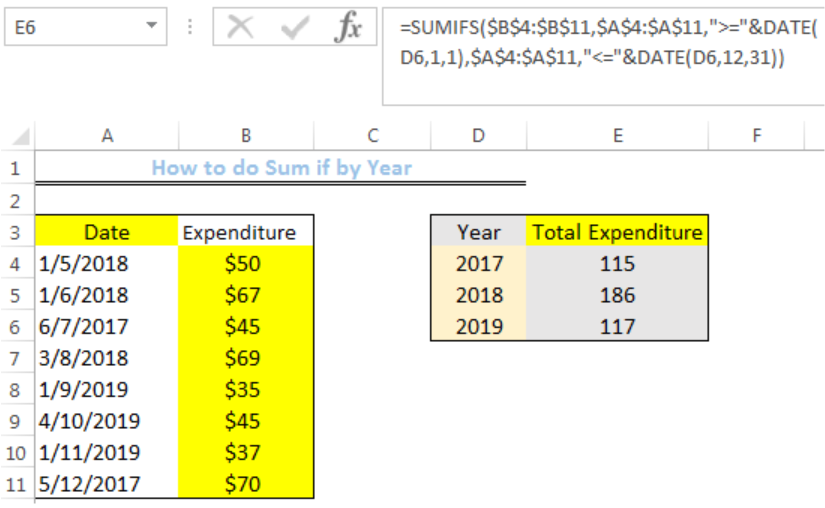Get instant live expert help with Excel or Google Sheets“My Excelchat expert helped me in less than 20 minutes, saving me what would have been 5 hours of work!”

#### Post your problem and you'll get expert help in seconds

Your message must be at least 40 characters
Our professional experts are available now. Your privacy is guaranteed.

# How to do Sumif by Year

We can use the SUMIFS Function to sum selected cells based on the values of the particular year we wish to add. The steps below will walk through the process.Figure 1: How to do Sum if by Year

## Syntax

`=SUMIFS(sum_range,date_range,”>=”&DATE(year,1,1),date_range,”<=”&DATE(year,12,31)`

## Formula

`=SUMIFS(\$B\$4:\$B\$11,\$A\$4:\$A\$11,">="&DATE(D4,1,1),\$A\$4:\$A\$11,"<="&DATE(D4,12,31))`

## Setting up the Data

• We will set up the data by inputting the Dates into Column A
• We will input the expenditure for each day in Column B
• Column D contains the years where expenditure was incurred
• Column E is where the formula will return the Total expenditure for 2017, 2018, and 2019Figure 2: Setting up the Data

## Sumif by Year

• We will click on Cell E4
• We will insert the formula below into the cell
`=SUMIFS(\$B\$4:\$B\$11,\$A\$4:\$A\$11,">="&DATE(D4,1,1),\$A\$4:\$A\$11,"<="&DATE(D4,12,31))`
• We will press the enter keyFigure 3: Sum of Expenditure for 2017

• We will click on Cell E4 again
• We will double-click on the fill handle (the small plus sign at the bottom right of Cell E4) and drag down to copy the formula into the other cellsFigure 4: Total Expenditure for 2017, 2018, and 2019

## Explanation

`=SUMIFS(\$B\$4:\$B\$11,\$A\$4:\$A\$11,">="&DATE(D4,1,1),\$A\$4:\$A\$11,"<="&DATE(D4,12,31))`

`=SUMIFS(sum_range,date_range,”>=”&DATE(year,1,1),date_range,”<=”&DATE(year,12,31)`

In this formula, the First DATE section is use to instruct that only cells greater than or equal to the DATE specified in Cell D4 are summed. This date is 2017/1/1, where 2017 is the year, 1 is the month, and the last 1 is the day.

For the Last Date section in the formula, it instructs that only cells lesser than or equal to the DATE specified in Cell D4 are summed. This date is 2017/12/31.

After meeting the criteria for the date_range (\$A\$4:\$A\$11), the values in the adjacent cells in Column B are searched for and summed.

## Instant Connection to an Expert through our Excelchat Service

Most of the time, the problem you will need to solve will be more complex than a simple application of a formula or function. If you want to save hours of research and frustration, try our live Excelchat service! Our Excel Experts are available 24/7 to answer any Excel question you may have. We guarantee a connection within 30 seconds and a customized solution within 20 minutes.

### Did this post not answer your question? Get a solution from connecting with the expert.Another blog reader asked this question today on Excelchat:## Subscribe to Excelchat.coAnother blog reader asked this question today on Excelchat: